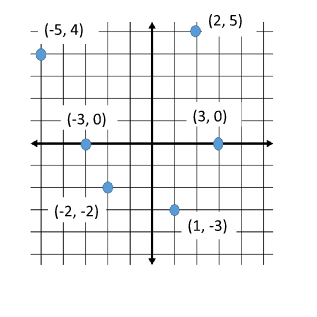# Graphing Points on a Coordinate Plane

The coordinate plane is formed by the x and y axis. The x and y axis cross each other at the point called the origin.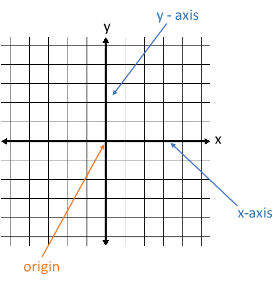Notice that when the x and y axis cross each other, the form four sections, called the quadrants. The quadrants are numbered in a counterclockwise direction. You can think of them forming a capital C.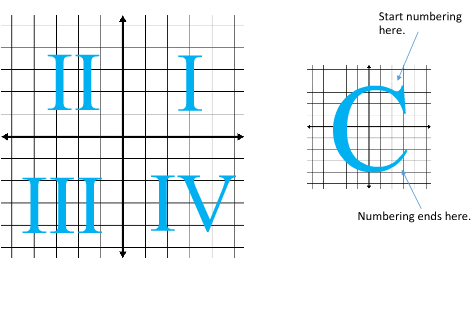The locations on the coordinate plane are written as coordinate pairs. We write the x value first and then the y. (x, y)

The point (3, 5) tells us to travel to the right three spaces from the origin and then up 5 spaces.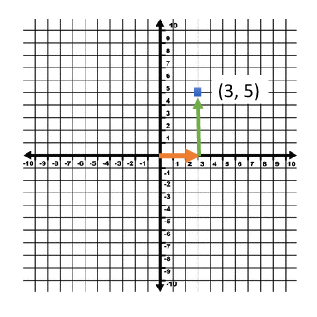Let's try another. (-4, -8)

We always start each point from the origin. We will move 4 to the left, because of the negative and then down 8.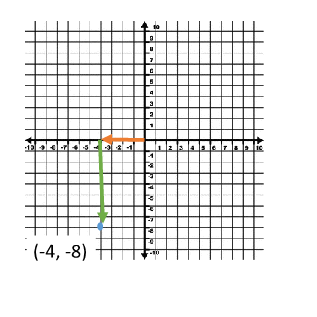Here are some tips for knowing where the point will be located:
- All of the points in the first quadrant will have a positive x and a positive y value. (+, +)

- All of the points in the second quadrant will have a negative x and a positive y value. ( -, +)

- All of the points in the third quadrant will have a negative x and a negative y value. ( - , -)

- All of the points in the fourth quadrant will have a positive x and a negative y value. (+, -)

Practice Naming Points: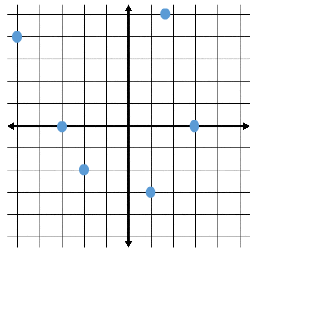When you go to name the points, be sure to always start from the origin. Then determine how far right or left you must go. This is the x value. Then you determine how far up or down you must go. This is the y value.